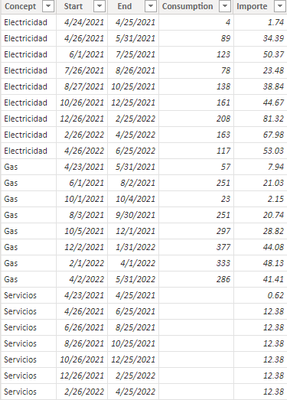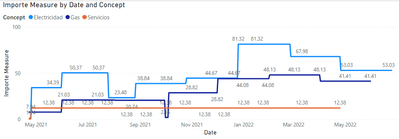cancel
Showing results for
Did you mean:## Convert start and end dates to continuous range

I have the following table:What is the best way to turn the Start and End columns into a date continuum so that you can easily graph the consumption and amounts per day?

1 ACCEPTED SOLUTIONCommunity Support

According to your description, I create a sample.Here's my solution.

1.Create a Date table, don't make relationship between the two tables.

``Date = CALENDARAUTO()``

2.Create two measures.

``````Consumption Measure =
MAXX (
FILTER (
'Table',
MAX ( 'Date'[Date] ) >= 'Table'[Start]
&& MAX ( 'Date'[Date] ) <= 'Table'[End]
),
'Table'[Consumption]
)
``````
``````Importe Measure =
MAXX (
FILTER (
'Table',
MAX ( 'Date'[Date] ) >= 'Table'[Start]
&& MAX ( 'Date'[Date] ) <= 'Table'[End]
),
'Table'[Importe]
)
``````

Put Date from Date table in X-axis, Concept in Legend and measure in Y-axis, get the result.I attach my sample below for your reference.

Best Regards,
Community Support Team _ kalyj

If this post helps, then please consider Accept it as the solution to help the other members find it more quickly.

7 REPLIES 7Community Support

According to your description, I create a sample.Here's my solution.

1.Create a Date table, don't make relationship between the two tables.

``Date = CALENDARAUTO()``

2.Create two measures.

``````Consumption Measure =
MAXX (
FILTER (
'Table',
MAX ( 'Date'[Date] ) >= 'Table'[Start]
&& MAX ( 'Date'[Date] ) <= 'Table'[End]
),
'Table'[Consumption]
)
``````
``````Importe Measure =
MAXX (
FILTER (
'Table',
MAX ( 'Date'[Date] ) >= 'Table'[Start]
&& MAX ( 'Date'[Date] ) <= 'Table'[End]
),
'Table'[Importe]
)
``````

Put Date from Date table in X-axis, Concept in Legend and measure in Y-axis, get the result.I attach my sample below for your reference.

Best Regards,
Community Support Team _ kalyj

If this post helps, then please consider Accept it as the solution to help the other members find it more quickly.Thank you very much for your answer, amazing the level of detail. I'm going to try to implement it and I tell you.Community Support

Is your problem solved? If so,would you mind accept the helpful replies as solutions? Then we are able to close the thread. More people who have the same requirment will find the solution quickly and benefit here, thank you!

Best Regards,
Community Support Team _ kalyjSuper User

Power query  can create date rows from your start and end date columns but how are those numbers supposed to be distributed? Are they going to be divided by the number of days from start to end so you'll get the daily amount?

Proud to be a Super User!

"Tell me and I’ll forget; show me and I may remember; involve me and I’ll understand."
Connect to me on LinkedIn || Need consulting? Hire me for a Power BI gig on UpWork.Yes, basically it is what I intend, to obtain a daily ratio, which can then be grouped to higher levels (monthly, annual), because it is impossible to know if one day there was more or less consumption. I simply know that for a certain period (the days between Start and End) there was X consumption that meant Y expense.Super User

This is sample M Script that generates dates using Start and End Dates columns.

``````let
Source = Table.FromRows(
Json.Document(
Binary.Decompress(
Binary.FromText(
"dZBBDoAgDAT/wtnEdhHavsX4/29IBJM14I2SSTu755m07qI7BJq21F6Cd6iWrq0BwYAzgA5kAuA0lOgAGGDa8wNA6U+FTtjCITNdSgcOlsy0wccG+5O0Om9AWZzgosBC7nMKOcghtG9g4EM7FgAbu8wpPj34qNr/JKssmhSOOSQ5OjizxaIHHqL1cN0=",
BinaryEncoding.Base64
),
Compression.Deflate
)
),
let
_t = ((type nullable text) meta [Serialized.Text = true])
in
type table [Start = _t, End = _t, Value = _t]
),
#"Changed Type" = Table.TransformColumnTypes(
Source,
{{"Start", type date}, {"End", type date}, {"Value", Int64.Type}}
),
#"Changed Type",
"Number of Days",
each Duration.Days([End] - [Start]) + 1,
Int64.Type
),
"Dates",
each List.Dates([Start], [Number of Days], #duration(1, 0, 0, 0)),
type list
),
#"Expanded Dates" = Table.ExpandListColumn(#"Added Custom1", "Dates"),
#"Changed Type1" = Table.TransformColumnTypes(#"Expanded Dates", {{"Dates", type date}}),
#"Changed Type1",
"New Value",
each [Value] / [Number of Days],
type number
),
#"Removed Other Columns" = Table.SelectColumns(
{"Start", "End", "Dates", "New Value"}
)
in
#"Removed Other Columns"``````

Proud to be a Super User!

"Tell me and I’ll forget; show me and I may remember; involve me and I’ll understand."
Connect to me on LinkedIn || Need consulting? Hire me for a Power BI gig on UpWork.